### Spherical Coordinates and the Angular Momentum Operators

The transformation from spherical coordinates to Cartesian coordinate is.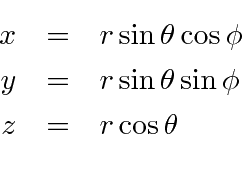The transformation from Cartesian coordinates to spherical coordinates is.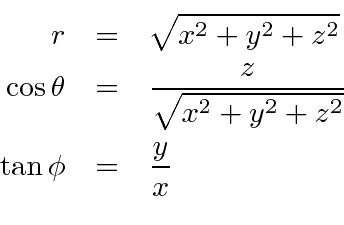We now proceed to calculate the angular momentum operators in spherical coordinates. The first step is to write the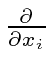in spherical coordinates. We use the chain rule and the above transformation from Cartesian to spherical. We have usedand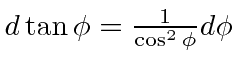. Ultimately all of these should be written in the sperical cooridnates but its convenient to usefor example in intermediate steps of the calculation.Bringing together the above results, we have.Now simply plug these into the angular momentum formulae.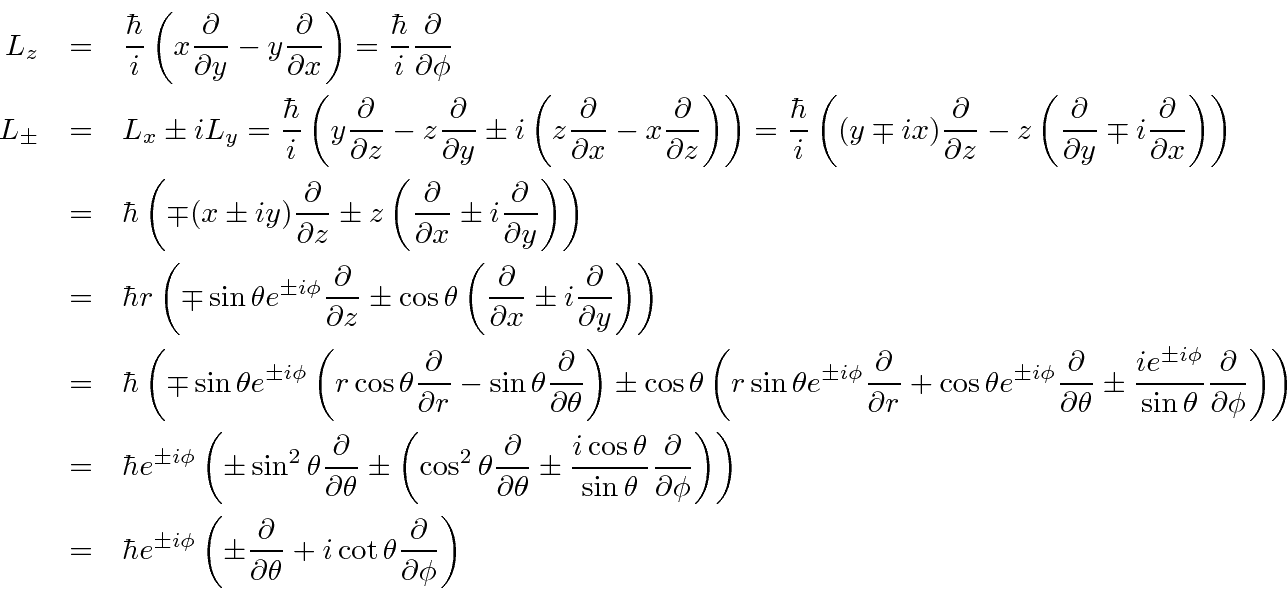We will use these results to find the actual eigenfunctions of angular momentum.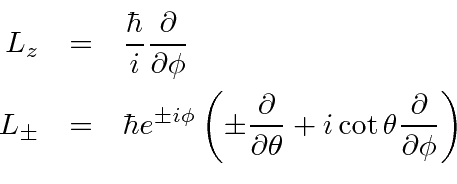Jim Branson 2013-04-22